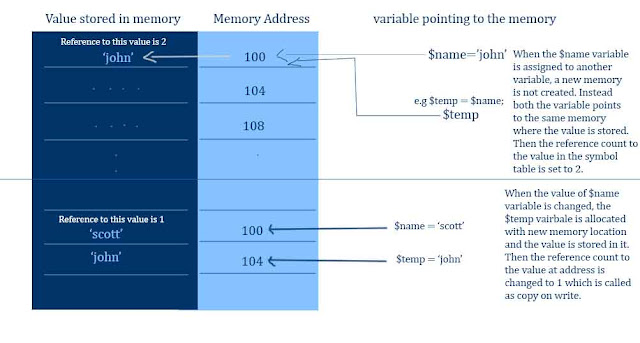PHP is a loose type language, in the sense that you don’t need to specify the variable type when you declare a variable. The variable takes the data type of the value that is assigned to it.

But how does it keeps track of the variable values and assignment of a variables value to another variable like this.

`\$variable1 = 5;\$variable2 = \$variable1;\$string_variable = 'This is a string';\$float_variable = 343.34;\$object_variable = new StdClass();`

PHP uses the Symbol table to keep track of variables values in the memory. PHP users reference counting and copy-on-write to manage the memory of storing the variables.

### Symbol Table

Symbol table is a method for storing the variable in the memory and also for efficient memory management. There are two parts for the symbol table,

• The name of the variable (i.e. \$name)
• The value of the variable itself. (i.e. ‘John’ which is stored in \$name)Small visualization that i made to represent the symbol table

### Reference Counting

A Symbol table is an array that maps variable names to the positions of their values in memory. When you copy a value from one variable to another, PHP dose not get more memory for a copy of the value to store it. Instead, it updates the symbol specifying that both the variables are pointing to the same part of memory.

#### Example

In the first part of the image show above \$temp is a copy of the \$name variable. Both the \$name and \$temp are pointing to the same address location in the memory i.e 100. The value that the memory location pointing to is ‘John’ and the number of reference to this 2 since \$name and \$temp are pointing to this.

`\$name = 'John'; // Stored at hex100 = 'John' - Reference count is 1\$temp = \$name; // Still Stored at hex 100 = 'John' - Reference count is 2;`

### Copy On Write

If you modify any one copy of the variable, then PHP allocates the required memory and makes the copy. This is called copy-on-write operation. The principle is that, when two variables are pointing to the same chunk of memory and when one variables value is modified only then is the copy of the assigned variable is created.

#### Example

From the above example, once the value of the \$name variable is changed then a new memory will be allocated for the ‘John’ and that memory address will be pointed to \$temp variable and reference count will become 1.

`\$name = 'scott'; // Stored at hex100 = 'Scott' - Reference count becomes 1\$temp = 'John'; // Allocated new memory address hex104 - Reference count is 1. `

The memory addresses shown above are just for explanatory purpose only. Original memory looks more different than this.